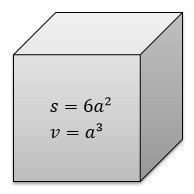Problems

# Surface area and volume 2

Time limit 1 second
Memory limit 128 MiBFind the surface area and volume of the cube by the length of its edge.

## Input data

The length of cube's edge a (a10^6).

## Output data

Print the surface area and volume of the cube .

## Examples

Input example #1
3

Output example #1
54 27

Source "ABC programming"